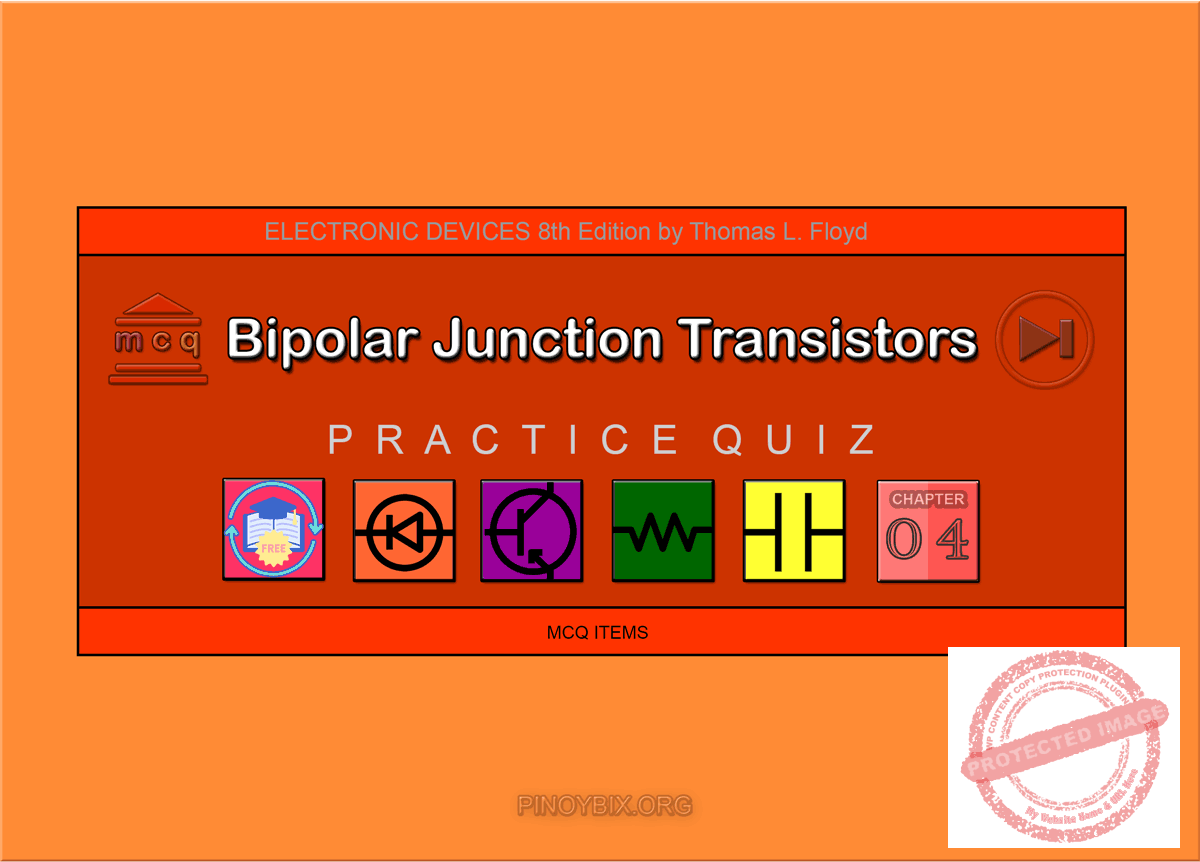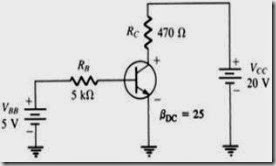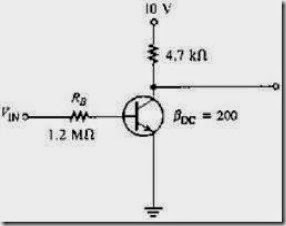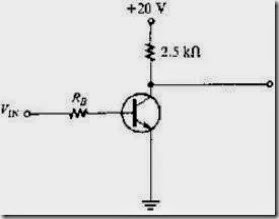# Floyd: MCQ in Bipolar Junction Transistors

(Last Updated On: November 9, 2020)This is the Multiple Choice Questions in Bipolar Junction Transistors from the book Electronic Devices – Electron Flow Version and Conventional Current Version 8th Edition by Thomas L. Floyd. If you are looking for a reviewer in Electronics Engineering this will definitely help. I can assure you that this will be a great help in reviewing the book in preparation for your Board Exam. Make sure to familiarize each and every questions to increase the chance of passing the ECE Board Exam.

#### Online Questions and Answers Topic Outline

• MCQ in Bipolar Junction Transistor (BJT) Structure
• MCQ in Basic BJT Operation
• MCQ in BJT Characteristics and Parameters
• MCQ in The BJT as an Amplifier
• MCQ in The Phototransistor
• MCQ in The BJT as a Switch
• MCQ in Transistor Categories and Packaging
• MCQ in GreenTech Application 4: Solar Power

If you are looking for the Self-test in Floyd’s Electronic Devices proceed to

#### Practice Exam Test Questions

Choose the letter of the best answer in each questions.

1. The dc load line on a family of collector characteristic curves of a transistor shows the

A) saturation region.

B) cutoff region.

C) active region.

D) all of the above

View Answer:

Answer: Option D

Solution:

2. A transistor data sheet usually identifies βDC as

A) hre.

B) hfe.

C) IC.

D) VCE.

View Answer:

Answer: Option B

Solution:

3. When a transistor is used as a switch, it is stable in which two distinct regions?

A) saturation and active

B) active and cutoff

C) saturation and cutoff

D) none of the above

View Answer:

Answer: Option C

Solution:

4. For a silicon transistor, when a base-emitter junction is forward-biased, it has a nominal voltage drop of

A) 0.7 V.

B) 0.3 V.

C) 0.2 V.

D) VCC.

View Answer:

Answer: Option A

Solution:

5. The value of βDC

A) is fixed for any particular transistor.

B) varies with temperature.

C) varies with IC.

D) varies with temperature and IC.

View Answer:

Answer: Option D

Solution:

6. The term BJT is short for

A) base junction transistor.

B) binary junction transistor.

C) both junction transistor.

D) bipolar junction transistor.

View Answer:

Answer: Option D

Solution:

7. A BJT has an IB of 50 µA and a βDC of 75; IC is:

A) 375 mA

B) 37.5 mA

C) 3.75 mA

D) 0.375 mA

View Answer:

Answer: Option C

Solution:

8. A certain transistor has IC = 15 mA and IB = 167 µA; βDC is:

A) 15

B) 167

C) 0.011

D) 90

View Answer:

Answer: Option D

Solution:

9. For normal operation of a pnp BJT, the base must be ______ with respect to the emitter and ______ with respect to the collector.

A) positive, negative

B) positive, positive

C) negative, positive

D) negative, negative

View Answer:

Answer: Option C

Solution:

10. A transistor amplifier has a voltage gain of 100. If the input voltage is 75 mV, the output voltage is:

A) 1.33 V

B) 7.5 V

C) 13.3 V

D) 15 V

View Answer:

Answer: Option B

Solution:

11. A 35 mV signal is applied to the base of a properly biased transistor with an r’e = 8 Ω and RC = 1 kΩ. The output signal voltage at the collector is:

A) 3.5 V

B) 28.57 V

C) 4.375 V

D) 4.375 mV

View Answer:

Answer: Option C

Solution:

12. Refer to Figure 4-1; the value of VBE is:Figure 4-1

A) 0.6 V

B) 0.7 V

C) 1.2 V

D) 0.079 V

View Answer:

Answer: Option B

Solution:

13. Refer to Figure 4-1; the value of VCE is:

A) 9.9 V

B) 9.2 V

C) 0.7 V

D) 19.3 V

View Answer:

Answer: Option A

Solution:

14. Refer to Figure 4-1; the value of VBC is:

A) 9.2 V

B) 9.9 V

C) –9.9 V

D) –9.2 V

View Answer:

Answer: Option D

Solution:

15. Refer to Figure 4-2; if VCE = 0.2 V, IC(sat) is:Figure 4-2

A) 0.05 mA

B) 2.085 mA

C) 1.065 mA

D) 7.4 mA

View Answer:

Answer: Option B

Solution:

16. Refer to Figure 4-2. Determine the minimum value of IB that will produce saturation.

A) 0.25 mA

B) 5.325 A

C) 1.065 A

D) 10.425 A

View Answer:

Answer: Option D

Solution:

17. Refer to Figure 4-2. Determine the minimum value of VIN from the following that will saturate this transistor.

A) 13.21 V

B) 12.51 V

C) 0.7 V

D) 9.4 V

View Answer:

Answer: Option A

Solution:

18. Refer to Figure 4-3. The value of βDC = 100 and VIN = 8 V. Determine IC(sat).Figure 4-3

A) 18 mA

B) 7.92 mA

C) 1.8 mA

D) 8 A

View Answer:

Answer: Option B

Solution:

19. Refer to Figure 4-3. In this circuit βDC = 100 and VIN = 8 V. The value of RB that will produce saturation is:

A) 92 kΩ

B) 9.1 MΩ

C) 100 kΩ

D) 150 kΩ

View Answer:

Answer: Option A

Solution:

20. Refer to Figure 4-3. The measured voltage, VCE, is 20 V. The transistor is in

A) saturation.

B) cutoff.

C) normal.

D) not enough data.

View Answer:

Answer: Option B

Solution:

21. Refer to Figure 4-3. You measure VCE and find it nearly equal to zero. You now know that the transistor is

A) operating in cutoff.

B) operating normally.

C) operating in saturation.

D) operating below cutoff.

View Answer:

Answer: Option C

Solution:

22. What is the order of doping, from heavily to lightly doped, for each region?

A) base, collector, emitter

B) emitter, collector, base

C) emitter, base, collector

D) collector, emitter, base

View Answer:

Answer: Option B

Solution:

23. What are the two types of bipolar junction transistors?

A) npn and pnp

B) pnn and nnp

C) ppn and nnp

D) pts and stp

View Answer:

Answer: Option A

Solution:

24. Which of the following is true for an npn or pnp transistor?

A) IE = IB + IC

B) IB = IC+ IE

C) IC = IB + IE

D) none of the above

View Answer:

Answer: Option A

Solution:

25. What is the ratio of IC to IB?

A) βDC

B) hFE

C) αDC

D) either βDC or hFE, but not αDC

View Answer:

Answer: Option D

Solution:

26. What is the ratio of IC to IE?

A) βDC

B) βDC / (βDC + 1)

C) αDC

D) either βDC / (βDC + 1) or αDC, but not βDC

View Answer:

Answer: Option D

Solution:

27. In what range of voltages is the transistor in the linear region of its operation?

A) 0 < VCE B) 0.7 < VCE < VCE(max) C) VCE(max) > VCE

D) none of the above

View Answer:

Answer: Option B

Solution:

28. What does DC vary with?

A) IC

B) °C

C) both IC and °C

D) IC’, but not °C

View Answer:

Answer: Option C

Solution:

29. What is (are) common fault(s) in a BJT-based circuit?

A) opens or shorts internal to the transistor

B) open bias resistor(s)

C) external opens and shorts on the circuit board

D) all of the above

View Answer:

Answer: Option D

Solution:

30. What is (are) general-purpose/small-signal transistors case type(s)?

A) TO-18

B) TO-92

C) TO-39

D) TO-52

E) all of the above

View Answer:

Answer: Option E

Solution:

31. The magnitude of dark current in a phototransistor usually falls in what range?

A) mA

B) μA

C) nA

D) pA

View Answer:

Answer: Option C

Solution:

### Complete List of Chapter Quiz in Floyd’s Electronic Devices

Please do Subscribe on YouTube!

P inoyBIX educates thousands of reviewers and students a day in preparation for their board examinations. Also provides professionals with materials for their lectures and practice exams. Help me go forward with the same spirit.

“Will you subscribe today via YOUTUBE?”

Subscribe